# KR100601689B1 - Method and apparatus for filtering section data - Google Patents

## Info

Publication number
KR100601689B1
KR100601689B1 KR1020040049744A KR20040049744A KR100601689B1 KR 100601689 B1 KR100601689 B1 KR 100601689B1 KR 1020040049744 A KR1020040049744 A KR 1020040049744A KR 20040049744 A KR20040049744 A KR 20040049744A KR 100601689 B1 KR100601689 B1 KR 100601689B1
Authority
KR
South Korea
Prior art keywords
value
bit
section
number
Prior art date
Application number
KR1020040049744A
Other languages
Korean (ko)
Other versions
KR20060000778A (en
Inventor
홍경표
Original Assignee
삼성전자주식회사
Priority date (The priority date is an assumption and is not a legal conclusion. Google has not performed a legal analysis and makes no representation as to the accuracy of the date listed.)
Filing date
Publication date
Application filed by 삼성전자주식회사 filed Critical 삼성전자주식회사
Priority to KR1020040049744A priority Critical patent/KR100601689B1/en
Publication of KR20060000778A publication Critical patent/KR20060000778A/en
Application granted granted Critical
Publication of KR100601689B1 publication Critical patent/KR100601689B1/en

• 238000001914 filtration Methods 0 title claims abstract description 71
• 230000001186 cumulative Effects 0 claims description 78
• 230000000875 corresponding Effects 0 claims description 21
• 239000002609 media Substances 0 claims description 12
• 238000009825 accumulation Methods 0 claims description 11
• 238000004364 calculation methods Methods 0 claims description 10
• 238000000034 methods Methods 0 description 1
• 239000000203 mixtures Substances 0 description 1

## Images

•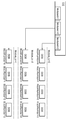•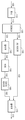•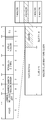•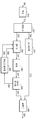•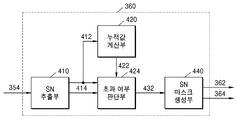•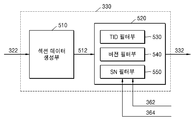•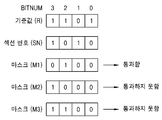•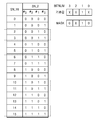•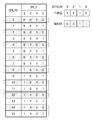•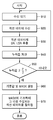## Classifications

• HELECTRICITY
• H04ELECTRIC COMMUNICATION TECHNIQUE
• H04NPICTORIAL COMMUNICATION, e.g. TELEVISION
• H04N21/00Selective content distribution, e.g. interactive television or video on demand [VOD]
• H04N21/40Client devices specifically adapted for the reception of or interaction with content, e.g. set-top-box [STB]; Operations thereof
• H04N21/43Processing of content or additional data, e.g. demultiplexing additional data from a digital video stream; Elementary client operations, e.g. monitoring of home network, synchronizing decoder's clock; Client middleware
• H04N21/434Disassembling of a multiplex stream, e.g. demultiplexing audio and video streams, extraction of additional data from a video stream; Remultiplexing of multiplex streams; Extraction or processing of SI; Disassembling of packetised elementary stream

## Abstract

A section data filtering method and apparatus are disclosed. The filtering apparatus according to the present invention includes a mask generation unit for generating a mask for use in filtering section data based on a section number in a digital broadcast receiver, Whether the mask is generated or not based on the accumulated value of the bit value 0 or the bit value 1 in the bitstream. According to the present invention, filtering is performed using the mask generated using the accumulated value of the received bit value, without directly comparing the section number of the section data received and stored in the receiver with the next received section number, Thereby reducing the filtering processing time.

## Description

METHOD AND APPARATUS FOR FILTERING SECTION DATA

FIG. 1A is a diagram showing a structure of digital broadcast data defined in ISO 13818-6; FIG.

2 is a diagram showing an internal structure of DDB and section data;

3 is a diagram showing a structure of a digital broadcast receiver according to the present invention;

4 is a diagram showing the internal structure of the mask generator 360 of FIG. 3 according to an embodiment of the present invention.

5 is a diagram showing the internal configuration of the filter unit 330;

6 is a view for explaining a filtering operation of a section number filter section;

Fig. 7 is a diagram showing section data that is discarded without being passed when the reference value is -1 and the mask is 0010; Fig.

Fig. 8 is a diagram showing section data discarded by the filter unit when the reference value is -10 and the mask is changed to 0011 in the state of Fig. 7. Fig.

9 is a time flow diagram illustrating a method of section number filtering according to the present invention.

The present invention relates to a method and apparatus for filtering section data of digital broadcasting, and more particularly, to a method and apparatus for filtering section data using a mask generated based on an accumulated value of each bit value of a section number.

The service data is provided from the broadcast provider to the receiver and then used in the microprocessor of the receiver to perform the above-described supplementary service. The service data includes data necessary for executing application programs and application programs produced using Java, HTML, MHEG, and the like. The service data enables the interactive service to be provided using the bidirectional channel. Examples of such interactive services include public opinion polls, popular vote polls, ratings polls, popular polls on broadcast figures, surveys, home shopping, and provision of production information for movies being broadcast.

1A is a diagram showing a structure of digital broadcast data defined in ISO 13818-6.

For digital media broadcasting, the prior art of data transmission protocols is the DSM-CC data carousel defined by ISO 13818-6. The data carousel groups data into logical units called modules and divides the modules into physical transmission units called blocks. The broadcast provider repeatedly transmits the block to the receiver. That is, the data carousel is repeatedly transmitted to the receiver through the transmission channel at regular intervals. The receiver receives the audio, video and service data block by block and reconstructs it as a module by assembling it. The module is used in the receiver as a logical unit for implementing the above-described service.

In order to realize such a transmission method, a data carousel defines two tables, a download data indicator (DDI) and a download data block (download data block). The DII has a module ID that identifies the module and a pointer to the DDB that carries the entity of the module. The transmitted module is divided into a certain size and then packed into the DDB for transmission. The DDB includes one or more section data (Section Data).

The digital broadcast receiver includes a tuner 110 for extracting a transport stream of a predetermined channel by selecting a channel desired by a user from among broadcast streams transmitted through a transport channel, a transport stream including audio / A demultiplexing unit 120 for dividing a stream 122 and a transport stream 124 including service data, a filter unit 140 for extracting user-desired section data from a transport stream including service data, A data processing unit 160 for reading the section data 152 stored in the buffer unit and generating a service output data 162 by processing a predetermined application, An AV processing unit 130 for generating AV output data 132 by processing a transport stream 122 including video data, The emitter 132 and service output data 162, an output unit 170 for output to the user.

According to ISO 13818-6, broadcast data transmitted in digital broadcasting is transmitted in the form of a transport stream. The transport stream contains one or more packets, and one packet has a fixed size of 188 Bytes. One packet ID (PID) is assigned to each packet, and the packet is classified into an audio packet, a video packet, and a data packet.

The filter unit 140 extracts only desired packets from the transport stream based on the packet ID, and then combines these to generate section data. The section data has a maximum size of 4096 Bytes. The section data forming one carousel data is one or more, and is transmitted to the receiver in blocks of a fixed size block called a download data block (DDB). That is, the DDB includes one or more section data.

2 is a diagram showing the internal structure of DDB and section data.

According to Fig. 2, the DDB includes L + 1 sections from section 0 to section L. The section data includes a section header and payload data.

Section headers include table ID, section length, table ID extension, version number, section number, last section number, and so on.

The table ID is an identifier specifying the DDB. The table ID extension is additional information added when the DDB can not be specified by the table ID, for example, a module ID may be included. The section number represents the serial number of the section within the DDB. For example, if the table ID extracted from an arbitrary section header is 0x3c, the section number is 0003, and the last section number is 000f, among the 16 section data included in the DDB whose table ID is 0x3c, Respectively. The version number indicates the version of the corresponding section data.

The filter unit 140 filters the section data desired by the user based on the table ID, the module ID, the version number, and the like. Here, the table ID and the like of the section data desired by the user are determined using PMT, AIT, DSI, DDI, or the like, which are various kinds of tables that the broadcasting provider transmits in advance to the receiver side.

The data processing unit 160 includes a memory (not shown) therein, and stores the section data transmitted from the buffer in the memory. The section data stored in the memory is used to execute the application by a separate processor in the data processing section.

However, according to the conventional receiver, when data loss occurs in the buffer and carousel data of a new period needs to be received, the section data stored in the buffer in the previous cycle is again stored in the buffer. That is, for example, when it is desired to receive carousel data including 16 section data from 0000 to 000f, section data from 0001 to 0004 is stored in the memory in the data processing section 160 in the first cycle, When the carousel data is received, the section data from 0001 to 0004 is stored in the buffer and then redundantly stored in the memory of the data processing section. This is because the determination as to whether or not the received section data is received in the previous period is not performed until reaching the data processing section.

This redundant storage of data increases the time required for complete transmission of carousel data required to execute one application due to the limited performance of the receiver and also consequently, It causes inconvenience to wait for a considerable time from input to output.

There is also a method of performing section data filtering by comparing the section number of the received section data with the section number of the section data already stored in the receiver in order to prevent the redundant storage of such data, The processing time is increased because all the bit values of the section number have to be compared, thereby increasing the time required for the filtering.

SUMMARY OF THE INVENTION Accordingly, the present invention has been made to solve the above-mentioned problems, and it is an object of the present invention to provide a method and apparatus for filtering a section data based on a section number using a mask generated based on an accumulated value of a bit value at each bit position of a section number already stored in a receiver And filtering the section data, thereby reducing the filtering time.

An apparatus for generating a mask for use in filtering section data based on a section number in a digital broadcast receiver, the apparatus comprising: means for determining a bit value 0 or a bit value 1 at each bit position of a section number already stored in the receiver And determines whether to generate the mask based on the accumulated value.

Further, the apparatus may further comprise: means for, when the cumulative value of 1 indicating the number of times the bit value 1 at the predetermined bit position of the section number is accumulated is equal to or greater than the total number of section data / 2, And generates the mask when the accumulated value of 0 indicating the accumulated number of bit values 0 is equal to or greater than the total number of section data / 2.

Here, the apparatus generates a reference value having a bit number equal to that of the mask and having a bit value of 1 at a bit position where the 0 cumulative value or the 1 cumulative value is greater than or equal to the total number of section data bits / 2 , Filtering is performed so that the section data having the section number is passed only when the bit value of the section number corresponding to the bit position where the bit value of the mask is 1 is different from the bit value of the reference value at the bit position.

The present invention also relates to a method for generating a mask for use in filtering section data based on a section number in a digital broadcast receiver, the method comprising the steps of: The generation of the mask is determined based on the accumulated value of the mask.

The present invention also relates to a method of filtering section data based on a section number in a digital broadcast receiver, the method comprising the steps of: a) filtering the section data based on a section number and a last section number of section data already stored in the digital broadcast receiver A mask generating step of generating a mask to be used; And b) a filtering step of filtering the section data based on the mask, wherein the mask generation step comprises: an accumulation value indicating the accumulated number of bit values at each bit position of the section number; And determining whether to generate the mask based on the number.

Hereinafter, a preferred embodiment of the present invention will be described in detail with reference to the accompanying drawings.

3 is a diagram illustrating a structure of a digital broadcast receiver according to the present invention.

A digital broadcast receiver according to the present invention includes a tuner unit 310 for extracting a transport stream of a predetermined channel by selecting a channel desired by a user from among broadcast streams transmitted through a transport channel, A demultiplexing unit 320 for demultiplexing the transport stream 322 including the service data and the transport stream 322 including the service data, a filter unit 330 for extracting the section data desired by the user from the transport stream including the service data, A buffer unit 340 for sequentially storing the extracted section data 332, a data processing unit 350 for generating the service output data 352 by processing the predetermined application by reading the section data 342 stored in the buffer unit An AV processing unit 37 for generating AV output data 372 by processing a transport stream 324 including audio / 0), the AV output data 372 and the service output data 352 to the user as an output signal 382 and the data from the data processing unit 350 to the data processing unit 350 in the previous cycle And a mask generator 360 for generating a reference value 362 and a mask value 364 on the basis of the received section number 354 of the section data and transmitting the generated reference value 362 and the mask value 364 to the filter unit 330.

The digital broadcast receiving apparatus shown in FIG. 3 performs filtering based on the fact that the mask generating unit 360 is added and the filter unit 330 based on the reference value 362 and the mask value 364 generated by the mask generating unit 360 And the like. This will be described in detail with reference to FIG. 4 and FIG.

FIG. 4 is a diagram showing the internal structure of the mask generator 360 of FIG. 3 according to an embodiment of the present invention.

The mask generation unit 360 includes a section number extraction unit 410, an accumulated value calculation unit 420, an excess determination unit 430, and a section number mask generation unit 440.

The section number extractor 410 receives the section data 354 of the carousel data transmitted in the previous cycle from the data processor 350 to the data processor 350 and receives the section number SN 412) and the last section number LSN (414).

The cumulative value calculation unit 420 receives the section number 412 from the section number extraction unit 410 and calculates the accumulated number of 0's and 1's at each bit position of the transmitted section number. Table 1 is a table showing the process in which cumulative value calculation is performed when the section number is 4 bits.

[Table 1]

Section number Bit 3 Bit 2 Bit 1 Bit 0 One 0 One 0 One 0 One 0 The first transmitted SN 1000 Y - - Y - Y - Y The second transmitted SN 1101 Y - Y - - Y Y - . . . Cumulative value for each bit 2 0 One One 0 2 One One

According to Table 1, each time the section number stored in the data processing unit 350 is input to the cumulative value calculation unit, the cumulative value calculation unit 420 calculates a cumulative value of '1' 0 &quot; is accumulated, that is, the 0 cumulative value for each bit is generated to generate the 1 cumulative value 424 and the 0 cumulative value 422. [ In the example of Table 1, 1 cumulative value C31 = 2 of bit 3, 0 cumulative value C30 = 0 of bit 3, 1 cumulative value C21 = 1 of bit 2, 0 cumulative value C31 = 1 of bit 2, 1 Cumulative value C11 = 0, 0 cumulative value of bit 1 C10 = 2, 1 cumulative value of bit 0 C01 = 1, 0 cumulative value of bit 0 C00 = 1.

The exceeding determination unit 430 determines whether the last section number 414 received from the section number extractor 410 and the 0 cumulative value 422 and 1 cumulative value 424 of each bit from the cumulative value calculator 420 ), It is determined whether or not section data that no longer needs to be received has occurred, and if the section data that no longer needs to be received has occurred, a command signal instructing the mask generating section 44 to generate a mask To the section number mask generation section 440. The section number mask generation section 440 outputs the mask generation command signal 432 to the section number mask generation section 440. [

A method of determining whether or not section data that the excessiveness determination unit 430 does not need to receive any more has occurred is as follows.

First, the number of total section data belonging to one carugel data is calculated based on the last section number. That is, the total number of section data = last section number + 1.

Then, based on the 0 cumulative value 422 or 1 cumulative value 424 received from the cumulative value calculation unit and the total number of section data calculated above, the section of section data that the broadcast receiving apparatus no longer needs to receive Check if the number exists. This step is performed by checking whether the accumulated value of 0 or 1 is greater than or equal to the total number of section data / 2 = P / 2.

Table 2 lists the 16 4-bit section numbers represented by binary numbers. According to Table 2, if the total number of section numbers is 16, the number of bit values 0 for each bit position of the section number indicated by the binary number is 8, and the number of 1 is 8. That is, according to the characteristics of the binary number, all the section data to be stored in the data processing section 350 includes the number of 0 or 1 existing at each bit position of the section number = the total number of all section numbers (the total number of section data) / 2 &lt; / RTI &gt; Therefore, if the accumulated value of 0 at a certain bit position is equal to or larger than the total number of section data / 2, it is determined that the section data corresponding to the section number having the bit value of the corresponding bit position is no longer required to be received It is possible. This embodiment uses the characteristics of this binary number to determine the section number to be filtered by the method described above.

[Table 2]

Section number_16 hexadecimal Section number_2 decimal 0 0000 One 0001 2 0010 3 0011 4 0100 5 0101 6 0110 7 0111 8 1000 9 1001 A 1010 B 1011 C 1100 D 1101 E 1110 F 1111

The mask generation information 432 includes an excess bit number N indicating a bit position where an accumulated value of 1 or 1 is exceeded and an excess bit value B indicating a bit value exceeded in the bit number. For example, if bit value 1 is exceeded in bit 3, then N = 3 and B = 1. The excess bit number N and the excess bit value B are generated based on the section number 412, the zero accumulation value and the one accumulation value 422,424.

The section number mask generation unit 440 generates the reference value 362 and the mask 364 based on the mask generation information 432 received from the excess determination unit 430. [ As described above, the mask generation information 432 includes an excess bit number N indicating a bit position where an accumulated value of 1 or 1 is exceeded, and an excess bit value B indicating a bit value exceeded in the bit number. The section number mask generation unit 400 generates a reference value and a mask according to the following reference value and mask definition.

The reference value 362 is a binary number having the same number of bits as the section number and the bit value at the bit position corresponding to the excess bit number N is the excess bit value B. [ The bit values at other bit positions are irrelevant.

The mask 364 also has the same number of bits as the section number, and is a binary number having a bit value of 1 at only the bit position corresponding to the excess bit number N and a bit value of 0 at the other bit positions. The role of the mask 364 in the filtering operation will be described later with reference to Figs. 5 and 6. Fig.

The present invention is characterized in that the filter unit 520 performs filtering using the mask and the reference value without comparing the section number of the section data stored in the data processing unit 350 with the section number directly received.

As a result, the specific bit value of the mask and the reference value described above is generated only when filtering is required, since the 0-cumulative value or 1-cumulative value is generated only when the cumulative value is equal to or greater than the total number of section data / 2.

In addition, instead of comparing all the bit values of the filtering section number, only the specific bit values determined by the mask and the reference value are compared, thereby significantly reducing the processing time of the filtering.

5 is a diagram showing the internal configuration of the filter unit 330. As shown in FIG.

The filter unit includes a section data generating unit 510 and a section data filter unit 520. The section data filter unit 520 includes a table ID filter unit 530, a version filter unit 540, and a section number filter unit 550 ).

The section data generation unit 510 receives the transport stream 322 including one or more packets, and then generates section data 512 by selectively combining the packets based on the packet ID. The generated section data 512 has the structure shown in FIG. 2 and includes a table ID, a version, a section number, and the like.

The section data filter unit 520 filters the section data based on the predetermined filtering information. The filtering information is information on which filtering is performed, and may be a table ID, a version, a section number, and the like. The section data filter unit 520 includes a table ID filter unit 530, a version filter unit 540, and a section number filter unit 550 according to the above-described filtering information.

According to a feature of the present invention, the section number filter unit 550 receives the reference value 362 and the mask 364 generated by the mask generation unit 360 as filtering reference information. The section number filter unit 550 performs the section number filtering by the reference value 362 and the mask 364. [

6 is a diagram for explaining the filtering operation of the section number filter unit.

The reference value R and the masks M1, M2, and M3 are the reference value 362 and the mask 364 transmitted from the mask generation unit 360. [ The section number SN is the section data input to the filter unit 330 or the section number of the section data filtered by the other filter units 530 and 540 in the section data filter unit. The bit number BitNum is an identifier that identifies the reference value, the section number, and the bit position of the mask.

When section data having a section number is received, the section number filter unit 550 sets the bit number of the section number existing at the bit position where the bit value of the mask (M1, M2, M3) Section data having the corresponding section number is passed only when the bit value of the reference value R is different from the bit value of the reference value R existing at the same bit position as the bit value. If there are more than two bit positions where the bit value of the mask is 1, the bit value of the reference value and the bit value of the section number should be different at every bit position.

In the example of FIG. 6, in the case of the mask M1, the bit position where the bit value of the mask M1 is 1 is BitNum = 2, and the bit value of the reference value is 1 and the bit value of the section number is 0 at this bit position. Since the bit value of the reference value differs from the bit value of the section number, the section data corresponding to the section number SN = 1010 is passed.

However, in the case of the mask M2, the bit position where the bit value of the mask M2 is 1 is BitNum = 3, and the bit value of the reference value is 1 and the bit value of the section number is also 1 at this bit position. Therefore, since the bit value of the reference value and the bit value of the section number are the same, the section data corresponding to the section number SN = 1010 can not pass and is discarded.

Finally, the case of the mask M3 will be described. The mask M3 has two bit positions with bit value 1. In the case of M3, the bit position where the bit value of the mask M3 is 1 is BitNum = 3,2, and the bit value of the reference value is 1,1 and the bit value of the section number is 1, 0 respectively. The bit value of the reference value at the bit position of BitNum = 2 differs from the bit value of the section number, but the bit value of the reference value at the bit position of BitNum = 3 is the same as the bit value of the section number, Is discarded without passing through.

As can be seen from the case of the mask M3, the section data that can not pass increases as the bit position where the bit value of the mask is 1 increases. When the number of section data stored in the data processing unit increases, a new mask is generated at a certain point and transferred to the filter unit. In this case, the new mask is a mask in which the bit position of the bit value 1 is increased by one. Herein, a certain point is a time point when 0 cumulative value or 1 cumulative value at a specific bit position becomes equal to or more than the number of section data / 2.

FIGS. 7 and 8 are diagrams showing the relationship between the bit position where the bit value of the mask is 1 and the change in the number of section data to be generated and filtered. FIG.

7 is a diagram showing section data which is discarded without being passed when the reference value is -1 and the mask is 0010. Fig. The meaning that the mask is 0010 and the reference value is -1- means that 1 cumulative value of the section data stored in the data processor is 8 or more at the bit number = 1. Since the bit position of the bit value 1 in the mask is bit number = 1, all the section data corresponding to the section number indicated by the block in Fig. 7 is discarded. That is, the binary section number of the discarded section data is 0010,0011,0110,0111,1010,1011,1110,1111, and the corresponding hexadecimal section number is 2,3,6,7,10,11,14,15 .

FIG. 8 is a diagram showing section data discarded by the filter unit when the reference value is -10 and the mask is changed to 0011 in the state of FIG. 7; FIG. The meaning that the reference value changes to -10 and the mask changes to 0010 means that in the state of Fig. 7, the cumulative value of 0 of the section data stored in the data processing section becomes 8 or more at the position of bit number = 0 .

According to the reference value and the mask in Fig. 8, a total of 8 + 4 = 12 pieces of section data can not be passed. Therefore, it is possible to prevent the twelve section data from being redundantly stored in the data processing unit.

9 is a time flow diagram illustrating a method of section number filtering according to the present invention.

In step 910, the section number filter unit 550 waits for section data to be received from the section data generation unit 510.

In step 920, the section number filter unit 550 receives the section data. The received section data may be section data filtered by combination with the operation of the other filter units 530 and 540 in the filter unit 330. [

In step 930, the section number extractor 410 extracts the section number SN and the last section number LSN from the section header of the section data.

In step 940, the cumulative value calculation unit 420 calculates a cumulative value of 1 and a cumulative value of 0 by counting the number of bit values 1 and 0 for each bit position of the section number each time a section number is received.

In step 950, the excess determination unit 430 determines whether the accumulated value of 1 or 0 calculated in step 940 is equal to or greater than the number of section data / 2 = P / 2. The number of sections can be generated from the last section number.

If greater than or equal to, proceed to step 960, otherwise proceed to step 910 to receive the next section data.

In step 960, a reference value and a mask are set by determining the bit value of the bit position where the 0 cumulative value or 1 cumulative value is larger than the number of section data / 2.

The reference value is set to 1 (when the accumulated value is 1) or 0 (when the accumulated value is 0) at the bit position where the accumulated value of 0 or 1 is larger than the number of section data / 2/2. The bit value may be set to any value.

The mask is set so that the bit value of the bit position where 0 cumulative value or 1 cumulative value is greater than the number of section data / 2 is 1 (both cumulative value and 0 cumulative value), and bit values of other bit positions are set to 0 . Herein, the mask value and mask value are generated by the mask generation unit 360, and then the mask value and the reference value are inputted to the section number filter unit 550 as filtering information.

In step 970, the section number filter unit 550 filters section data based on the mask and reference value set in step 960.

When section data having a section number is received, the section number filter unit 550 sets the bit number of the section number existing at the bit position of the bit value 1 in the mask to the bit position Section data having the corresponding section number is passed only when the bit value of the reference value is different from the bit value of the reference value existing in the section number. If there are more than two bit positions where the bit value of the mask is 1, the bit value of the reference value and the bit value of the section number should be different at every bit position.

When the broadcast receiver receives the carugel data of the first period, since no overlapping section data occurs in one carugel data, only the loop of FIG. 9, that is, steps 910 to 950 will be performed. However, when a broadcast receiver receives a new period carousel data due to a loss of section data due to a difference in processing speed between the buffer and the data processing unit or for other reason, redundant section data may be generated, and this section data is stored in steps 960 to 970 Lt; / RTI &gt;

Meanwhile, the section number filtering method according to the present invention can be written in a computer program. The codes and code segments that make up the program can be easily deduced by a computer programmer in the field. In addition, the program is stored in computer readable media readable by a computer, and is read and executed by a computer to implement section number filtering. The information storage medium includes a magnetic recording medium, an optical recording medium, and a carrier wave medium.

The present invention has been described with reference to the preferred embodiments. It will be understood by those skilled in the art that various changes in form and details may be made therein without departing from the spirit and scope of the invention as defined by the appended claims. Therefore, the disclosed embodiments should be considered in an illustrative rather than a restrictive sense. The scope of the present invention is defined by the appended claims rather than by the foregoing description, and all differences within the scope of equivalents thereof should be construed as being included in the present invention.

As described above, according to the method and apparatus for filtering section data according to the present invention, the section number of the section data already received and stored in the receiver is not directly compared with the next received section number, and the accumulated value of the received bit value is used And the filtering is performed using the generated mask, thereby reducing the overall processing time.

Further, instead of comparing all the bit values of the section number at the time of filtering, only the specific bit values determined by the mask and the reference value are compared, and the processing time of the filtering can be remarkably reduced.

## Claims (37)

1. An apparatus for generating a mask for use in filtering section data based on a section number in a digital broadcast receiver,
And determines whether to generate the mask based on the accumulated value of bit value 0 or bit value 1 at each bit position of the section number already stored in the receiver.
2. 2. The apparatus of claim 1,
The cumulative value of 1 indicating the number of times the bit value 1 at the predetermined bit position of the section number is accumulated is greater than or equal to the total number of section data / 2
If the accumulated value of 0 indicating the number of times the bit value 0 is accumulated at the predetermined bit position of the section number is equal to or greater than the total number of section data / 2
3. The apparatus of claim 2, wherein the apparatus has a bit number equal to that of the mask, and the bit value at a bit position where the 0 cumulative value or the 1 cumulative value is greater than or equal to the total number of section data / A reference value is generated,
Only when the bit value of the section number corresponding to the bit position where the bit value of the mask is 1 is different from the bit value of the reference value at the bit position, .
4. 4. The apparatus of claim 3, wherein two or more bit positions with a bit value of 1 in the mask are two or more,
Wherein filtering is performed so that section data having the section number is passed only when the bit value of the reference value and the bit value of the section number are different at every bit position where the bit value of the mask is 1.
5. 2. The apparatus of claim 1,
a) a section number extracting unit for extracting a section number and a last section number from section data already stored in the digital broadcast receiver;
b) an accumulation value calculation unit for receiving the section number and calculating an accumulated value of 0, which is a value indicative of the cumulative count of the bit value 0 and a cumulative value of 1 indicating a cumulative count of the bit value 1 at each bit position of the section number; ;
c) an excess determination unit for determining whether to generate the mask based on the 0 accumulation value and the 1 accumulation value; And
d) generating a mask based on a bit position exceeding the 0 cumulative value or the 1 cumulative value in the section number when it is determined to generate the mask,
&Lt; / RTI &gt;
6. The apparatus of claim 4, wherein the section number mask generator comprises:
And generates a mask in which the bit value of the excess bit position is one and the other bit value is zero.
7.  6. The apparatus of claim 5, wherein the section number mask generation unit further generates a reference value having a bit value of 1 at the bit position exceeding 1 when the bit value at the excess bit position of the section number is 1,
Only when the bit value of the section number corresponding to the bit position where the bit value of the mask is 1 is different from the bit value of the reference value at the bit position, .
8. An apparatus for filtering section data based on a section number in a digital broadcast receiver,
a) a mask generator for generating a mask used for filtering the section data based on a section number and a last section number of the section data already stored in the digital broadcast receiver; And
b) a filter unit for filtering the section data based on the mask,
Wherein the mask generation unit determines whether to generate the mask based on the cumulative value indicating the cumulative number of bit values at each bit position of the section number and the total number of section data.
9. 9. The apparatus according to claim 8,
If the accumulated value of 1 indicating the number of times of accumulation of the bit value 1 at each bit position of the section number is equal to or greater than the total number of section data / 2
Or when the accumulated value of 0 indicating the number of times the bit value 0 is accumulated at each bit position of the section number is greater than or equal to the total number of section data /
10. The apparatus as claimed in claim 9, wherein the mask generator has a bit number equal to that of the mask, and the 0 cumulative value or the 1 cumulative value has a bit value of 1 at a bit position equal to or greater than the total number of section data / , Generates a reference value,
The filter unit passes section data having the section number only when the bit value of the section number corresponding to the bit position of the bit of the mask is different from the bit value of the reference value at the bit position .
11. 11. The method of claim 10, wherein the bit positions of the mask have bit positions of 1 and 2,
Wherein the filter unit passes the section data having the section number only when the bit value of the reference value and the bit value of the section number are different at every bit position where the bit value of the mask is 1.
12. 9. The apparatus according to claim 8,
a1) a section number extractor for extracting a section number and a last section number from the section data already stored in the digital broadcast receiver;
a2) an accumulation value calculator receiving the section number and calculating an accumulated value of a bit value of 1 at each bit position of the section number and an accumulated value of a bit value of 0;
a3) an excess determination unit for determining whether to generate the mask based on the 0 accumulation value and the 1 accumulation value; And
a4) If it is determined to generate a mask, the mask is set based on the excess bit number indicating the bit position at which the 0 cumulative value or 1 cumulative value has been exceeded in the section number and an excess bit value indicating a bit value exceeding that bit number And generating a section number mask generating section.
13. 13. The apparatus of claim 12, wherein the section number mask generator comprises:
When the accumulated value of 1 indicating the number of times the bit value 1 at the predetermined bit position of the section number is accumulated is equal to or greater than the total number of section data /
Or when the accumulated value of 0 indicating the number of times the bit value 0 is accumulated at the predetermined bit position of the section number is greater than or equal to the total number of section data /
14. 11. The filter according to claim 10,
b1) a section data generator for generating the section data from the transport stream transmitted to the digital broadcasting receiver; And
b2) a section data filter section for filtering the section data based on filtering information identifying section data necessary for execution of the application requested by the user;
&Lt; / RTI &gt;
15. 15. The apparatus of claim 14, wherein the filtering information is one of a table ID, a version, and a section number.
16. 15. The apparatus of claim 14,
b21) section number filter section for filtering the section data based on the section number.
17. The apparatus as claimed in claim 16, wherein the section number filter unit stores the section number only when the bit value of the section number corresponding to the bit position where the bit value of the mask is 1 is different from the bit value of the reference value at the bit position Wherein the first section passes the section data.
18. 18. The apparatus of claim 17, wherein the bit positions of the mask are two or more, and the filter unit is configured to perform a mask operation in which the bit value of the reference value and the bit value of the section number are different Only section data having the section number is passed.
19. A method of generating a mask for use in filtering section data based on a section number in a digital broadcast receiver,
Wherein the generation of the mask is determined based on an accumulated value of a bit value 0 or a bit value 1 at each bit position of a section number already stored in the receiver.
20. 20. The method of claim 19,
The cumulative value of 1 indicating the number of times the bit value 1 at the predetermined bit position of the section number is accumulated is greater than or equal to the total number of section data / 2
If the accumulated value of 0 indicating the number of times the bit value 0 is accumulated at the predetermined bit position of the section number is equal to or greater than the total number of section data / 2
21. 21. The method of claim 20,
Generating a reference value having a bit number equal to the mask and having a bit value of 1 at a bit position where the 0 cumulative value or the 1 cumulative value is greater than or equal to the total number of section data bits /
Only when the bit value of the section number corresponding to the bit position where the bit value of the mask is 1 is different from the bit value of the reference value at the bit position, Lt; / RTI &gt;
22. 22. The apparatus of claim 21, wherein the bit positions of the mask have bit positions of 1 and 2,
Wherein the filtering is performed so that the section data having the section number is passed only when the bit value of the reference value and the bit value of the section number are different at every bit position where the bit value of the mask is 1. [
23. 20. The method of claim 19,
a) a section number extracting step of extracting a section number and a last section number from section data already stored in the digital broadcast receiver;
b) a cumulative value calculation step of receiving the section number and calculating a cumulative value of 1, which is a value indicating the cumulative number of bit values 1 at each bit position of the section number, and a cumulative value of 0, ;
c) determining whether to generate the mask based on the 0 cumulative value and the 1 cumulative value; And
d) a section number mask generation step of generating a mask based on a bit position exceeding the 0 cumulative value or the 1 cumulative value in the section number when it is determined to generate the mask;
&Lt; / RTI &gt;
24. 23. The method of claim 22, wherein the step of generating the section number mask comprises:
And generating a mask in which the bit value of the excess bit position is one and the other bit value is zero.
25.  24. The method of claim 23, wherein the step of generating the section number mask comprises: generating a reference value having a bit value of 1 at the excess bit position when the bit value at the excess bit position of the section number is 1 Further included,
Only when the bit value of the section number corresponding to the bit position where the bit value of the mask is 1 is different from the bit value of the reference value at the bit position, Lt; / RTI &gt;
26. A method of filtering section data based on a section number in a digital broadcast receiver,
a) generating a mask used for filtering the section data based on a section number and a last section number of section data already stored in the digital broadcast receiver; And
b) filtering the section data based on the mask,
The mask generation step includes the step of determining whether to generate the mask based on the cumulative value indicating the cumulative number of bit values at each bit position of the section number and the total number of section data How to.
27. 27. The method of claim 26,
If the accumulated value of 1 indicating the number of times of accumulation of the bit value 1 at each bit position of the section number is equal to or greater than the total number of section data / 2
Or when the accumulated value of 0 indicating the number of times the bit value 0 is accumulated at each bit position of the section number is greater than or equal to the total number of section data /
&Lt; / RTI &gt; wherein the step of generating the mask comprises generating the mask.
28. 28. The method as claimed in claim 27, wherein the mask generation step has a bit number equal to that of the mask, and when the bit value at the bit position where the 0 cumulative value or the 1 cumulative value is equal to or greater than the total number of section data / And generating a reference value,
The filtering step comprises passing the section data having the section number only when the bit value of the section number corresponding to the bit position of the bit of the mask is different from the bit value of the reference value at the bit position &Lt; / RTI &gt;
29. 29. The method of claim 28, wherein the bit positions of the mask have bit positions of 1 and 2,
The filtering step may further include passing section data having the section number only when the bit value of the reference value and the bit value of the section number are different at every bit position where the bit value of the mask is 1 Lt; / RTI &gt;
30. 27. The method of claim 26,
a1) extracting a section number and a last section number from the section data already stored in the digital broadcast receiver;
a2) a cumulative value calculation step of receiving the section number and calculating an accumulated value of 1 representing cumulative number of bit values 1 at each bit position of the section number and an accumulated value of 0 representing cumulative number of bit values of 0;
a3) determining whether to generate the mask based on the 0 cumulative value and the 1 cumulative value; And
a4) If it is determined to generate a mask, the mask is set based on the excess bit number indicating the bit position at which the 0 cumulative value or 1 cumulative value has been exceeded in the section number and an excess bit value indicating a bit value exceeding that bit number And generating a section number mask to be generated.
31. The method of claim 30, wherein the step of generating the section number mask comprises:
When the accumulated value of 1 indicating the number of times the bit value 1 at the predetermined bit position of the section number is accumulated is equal to or greater than the total number of section data / 2
Or when the accumulated value of 0 indicating the number of times the bit value 0 is accumulated at the predetermined bit position of the section number is greater than or equal to the total number of section data /
&Lt; / RTI &gt; wherein the step of generating the mask comprises generating the mask.
32. 29. The method of claim 28,
b1) a section data generation step of generating the section data from a transport stream transmitted to the digital broadcasting receiver; And
b2) a section data filtering step of filtering the section data based on filtering information identifying section data necessary for the execution of the application requested by the user
&Lt; / RTI &gt;
33. 33. The method of claim 32, wherein the filtering information is one of a table ID, a version, and a section number.
34. 33. The method of claim 32,
b21) a section number filtering step of filtering the section data based on the section number.
35. 35. The method of claim 34, wherein the filtering of the section number is performed only when the bit value of the section number corresponding to the bit position where the bit value of the mask is 1 is different from the bit value of the reference value at the bit position, &Lt; / RTI &gt; comprising passing section data having a plurality of data segments.
36. 36. The method of claim 35, wherein if the bit number of the mask is two or more, the step of filtering the section number comprises: comparing the bit value of the reference value with the bit number of the section number And passing section data having the section number only if the bit values are different.
37. A computer-readable recording medium storing a program for causing a computer to execute the method according to claim 19.
KR1020040049744A 2004-06-29 2004-06-29 Method and apparatus for filtering section data KR100601689B1 (en)

## Priority Applications (1)

Application Number Priority Date Filing Date Title
KR1020040049744A KR100601689B1 (en) 2004-06-29 2004-06-29 Method and apparatus for filtering section data

## Applications Claiming Priority (4)

Application Number Priority Date Filing Date Title
KR1020040049744A KR100601689B1 (en) 2004-06-29 2004-06-29 Method and apparatus for filtering section data
JP2005188781A JP4805616B2 (en) 2004-06-29 2005-06-28 Section data filtering method and apparatus
EP05254041A EP1613057A1 (en) 2004-06-29 2005-06-29 Method and apparatus for filtering section data
US11/169,061 US20050286563A1 (en) 2004-06-29 2005-06-29 Method and apparatus for filtering section data

## Publications (2)

Publication Number Publication Date
KR20060000778A KR20060000778A (en) 2006-01-06
KR100601689B1 true KR100601689B1 (en) 2006-07-14

# Family

## Family Applications (1)

Application Number Title Priority Date Filing Date
KR1020040049744A KR100601689B1 (en) 2004-06-29 2004-06-29 Method and apparatus for filtering section data

## Country Status (4)

US (1) US20050286563A1 (en)
EP (1) EP1613057A1 (en)
JP (1) JP4805616B2 (en)
KR (1) KR100601689B1 (en)

## Families Citing this family (8)

* Cited by examiner, † Cited by third party
Publication number Priority date Publication date Assignee Title
JP4825460B2 (en) * 2005-06-29 2011-11-30 東芝デジタルメディアエンジニアリング株式会社 Receiving apparatus and receiving method
US7826712B2 (en) * 2006-08-29 2010-11-02 Echostar Technologies Corporation Method and apparatus for receiving, storing, and presenting multimedia programming without indexing prior to storage
JP4912856B2 (en) * 2006-12-15 2012-04-11 東芝デジタルメディアエンジニアリング株式会社 Filtering device and digital broadcast receiving device
US8645400B1 (en) * 2008-08-01 2014-02-04 Marvell International Ltd. Flexible bit field search method
US20110145837A1 (en) * 2009-12-14 2011-06-16 Bower Kenneth S Filtering Broadcast Recipients In A Multiprocessing Environment
CN102591791B (en) * 2011-12-31 2016-08-03 深圳市中兴昆腾有限公司 A kind of system and method for reducing data storage capacity by defining strategy
US10387492B2 (en) * 2014-05-12 2019-08-20 Rakuten, Inc. Information processing system, information processing method, and program

## Citations (4)

* Cited by examiner, † Cited by third party
Publication number Priority date Publication date Assignee Title
WO1998043415A1 (en) * 1997-03-21 1998-10-01 Canal+ Societe Anonyme Extracting data sections from a transmitted data stream
KR20000050774A (en) * 1999-01-14 2000-08-05 구자홍 Method for PSI filtering
KR20010086911A (en) * 2000-03-04 2001-09-15 구자홍 Section filtering method
KR20020014958A (en) * 2000-08-19 2002-02-27 박종섭 PSI data filtering device for MPEG-2 system and control method thereof

## Family Cites Families (11)

* Cited by examiner, † Cited by third party
Publication number Priority date Publication date Assignee Title
US4204193A (en) * 1978-11-03 1980-05-20 International Business Machines Corporation Adaptive alignment for pattern recognition system
US5127014A (en) * 1990-02-13 1992-06-30 Hewlett-Packard Company Dram on-chip error correction/detection
WO1999059290A1 (en) * 1998-05-13 1999-11-18 Sony Corporation System for providing contents
JP3809278B2 (en) * 1998-07-02 2006-08-16 松下電器産業株式会社 Multiple packet separator
US6636881B1 (en) * 1999-01-18 2003-10-21 Matsushita Electric Industrial Co., Ltd. Binary data counter, area information extractor and huffman converter
JP2001053700A (en) * 1999-08-05 2001-02-23 Matsushita Electric Ind Co Ltd Data selecting device and data processor
JP2001308812A (en) * 2000-04-24 2001-11-02 Nec Microsystems Ltd Method for separation processing of transport stream for digital television and its recording medium
EP1267579A3 (en) * 2001-06-11 2003-03-19 Canal+ Technologies Société Anonyme MPEG table structure
US7116663B2 (en) * 2001-07-20 2006-10-03 Pmc-Sierra Ltd. Multi-field classification using enhanced masked matching
US7025515B2 (en) * 2003-05-20 2006-04-11 Software 2000 Ltd. Bit mask generation system
JP4176044B2 (en) * 2004-05-20 2008-11-05 東芝デジタルメディアエンジニアリング株式会社 Filtering device and digital broadcast receiving device

## Patent Citations (4)

* Cited by examiner, † Cited by third party
Publication number Priority date Publication date Assignee Title
WO1998043415A1 (en) * 1997-03-21 1998-10-01 Canal+ Societe Anonyme Extracting data sections from a transmitted data stream
KR20000050774A (en) * 1999-01-14 2000-08-05 구자홍 Method for PSI filtering
KR20010086911A (en) * 2000-03-04 2001-09-15 구자홍 Section filtering method
KR20020014958A (en) * 2000-08-19 2002-02-27 박종섭 PSI data filtering device for MPEG-2 system and control method thereof

## Also Published As

Publication number Publication date
JP4805616B2 (en) 2011-11-02
KR20060000778A (en) 2006-01-06
JP2006020310A (en) 2006-01-19
EP1613057A1 (en) 2006-01-04
US20050286563A1 (en) 2005-12-29

## Similar Documents

Publication Publication Date Title
US20180376100A1 (en) Receiving device, receiving method, transmitting device, and transmitting method
US20170264933A1 (en) Contents reception device and method, contents transmission device and method, program, and recording medium
US10244287B2 (en) Transmission apparatus and method, reception apparatus and method, and transmission and reception system
EP2624548B1 (en) Receiving device, receiving method, and program
KR101378088B1 (en) Computer implemented method and contents receiving apparatus using an electronic questionnaire for advanced services
US8875169B2 (en) Transmission and reception apparatus, methods, and systems for filtering content
JP6408762B2 (en) Receiving apparatus, receiving method, and program
KR100609337B1 (en) Apparatus, storage medium and method for forming and processing program specific information containing text data for terrestrial, cable or satellite broadcast
AU737150B2 (en) A system for forming and processing text data for use in program specific information for broadcast
EP0933926B1 (en) DMA controller
DE69935360T2 (en) Electronic program guide and corresponding MPEG data stream
KR100684658B1 (en) Apparatus and method for forming program guide infomation
CN101267524B (en) Method for retrieving lost content for a scheduled program and digital video recorder
US20160277788A1 (en) Transmission method for broadcast service, reception method therefor, and reception apparatus therefor
EP2613267A1 (en) Reception device, reception method, transmission device, transmission method, program, and broadcast system
KR101486357B1 (en) method of transmitting and receiving a broadcasting signal and apparatus for receiving a broadcasting signal
US8752115B2 (en) System and method for aggregating commercial navigation information
KR100910878B1 (en) A transmission system comprising a transmitter and at least one receiver configured to receive signals transmitted therefrom
JP5214659B2 (en) Transport stream for program stream conversion
AU761080B2 (en) A system for forming and processing program map information suitable for terrestrial, cable or satellite broadcast
EP0758833B1 (en) Method and apparatus for providing an interactive guide to events available on an information network
AU770163B2 (en) System and method for recording pushed data

## Legal Events

Date Code Title Description
A201 Request for examination
E902 Notification of reason for refusal
E701 Decision to grant or registration of patent right
GRNT Written decision to grant
FPAY Annual fee payment

Payment date: 20130627

Year of fee payment: 8

FPAY Annual fee payment

Payment date: 20140627

Year of fee payment: 9

FPAY Annual fee payment

Payment date: 20150629

Year of fee payment: 10

FPAY Annual fee payment

Payment date: 20160629

Year of fee payment: 11

FPAY Annual fee payment

Payment date: 20170629

Year of fee payment: 12

LAPS Lapse due to unpaid annual fee